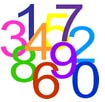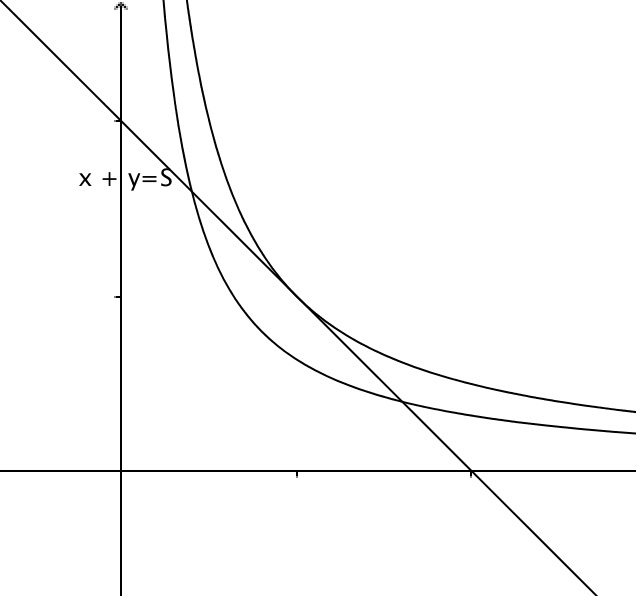Various pairs of numbers add together to give a sum of 10:

3 + 7 = 10
2 + 8 = 10
4.4 + 5.6 = 10

The products of these numbers are all different:

3 x 7 = 21
2 x 8 = 16
4.4 x 5.6 = 24.64

What is the greatest product that can be made from pairs of numbers that add up to 10?

Which of the rectangles that have a perimeter of 20 units has the greatest area?

Try using different starting numbers.

Can you find a strategy for splitting numbers so that you always get the greatest product?How does this graph show the greatest product of two numbers that add up to a fixed sum?

How would you use calculus to find the greatest product of pairs of numbers that add up to any given sum?

Does this result for two numbers generalise to triples of numbers that add up to a given sum?

Tagged with: